Wang, Y., Fei, R., Feng, C., and Fang, S. (2020). "Failure modes of insulating pressboard subjected to high electric fields and durations: Evidence of partial discharge and changes at the microscopic scale," BioRes. 15(2), 3585-3603.

#### Abstract

The deterioration of insulation pressboard under needle-plate discharge was tested, and the degradation stage was divided according to observed experimental phenomena. Based on the improved Top-hat watershed image segmentation method, the white marks area of the pressboard were tested. Fibres with different discharge states were extracted from SEM images. The fibre width was calculated, and the porosity of the surface of the insulation pressboard at different degradation stages was calculated. Simultaneously, the 3D reconstruction technique was used to observe the 3D morphology of fibres at different discharge stages. The study found that with the deepening of the discharge process, the discharge of the pressboard increased, and the white marks of the pressboard continued to expand from point to surface. In addition, the diameter of the fibre of the insulating pressboard decreased obviously with the increase of the pressing time, and the fibre diameter was 89.6% after the breakdown. Moreover, the electrical stress had a great effect on the expansion of the interlayer pores of the pressboard; the cross-section porosity of the insulating pressboard gradually increased with the deepening of the discharge process, and after breakdown, the interlayer porosity reached 12.5%.

Failure Modes of Insulating Pressboard Subjected to High Electric Fields and Durations: Evidence of Partial Discharge and Changes at the Microscopic Scale

Yongqiang Wang,* Ruoyu Fei, Changhui Feng, and Sen Fang

The deterioration of insulation pressboard under needle-plate discharge was tested, and the degradation stage was divided according to observed experimental phenomena. Based on the improved Top-hat watershed image segmentation method, the white marks area of the pressboard were tested. Fibres with different discharge states were extracted from SEM images. The fibre width was calculated, and the porosity of the surface of the insulation pressboard at different degradation stages was calculated. Simultaneously, the 3D reconstruction technique was used to observe the 3D morphology of fibres at different discharge stages. The study found that with the deepening of the discharge process, the discharge of the pressboard increased, and the white marks of the pressboard continued to expand from point to surface. In addition, the diameter of the fibre of the insulating pressboard decreased obviously with the increase of the pressing time, and the fibre diameter was 89.6% after the breakdown. Moreover, the electrical stress had a great effect on the expansion of the interlayer pores of the pressboard; the cross-section porosity of the insulating pressboard gradually increased with the deepening of the discharge process, and after breakdown, the interlayer porosity reached 12.5%.

Keywords: Insulation pressboard; Image processing; Fibre; Scanning electron microscope

Contact information: Hebei Provincial Key Laboratory of Power Transmission Equipment Security, Department of Electrical Engineering, North China Electric Power University, Baoding 071003, China;

*Corresponding author: qianghd@126.com

INTRODUCTION

The power transformer is an important part of transmission and distribution equipment for power systems, and partial discharge is one of the causes of insulation damage in electrical equipment (Tatsuki et al. 2017). However, in the process of transformer production, transportation, and operation, insulation materials often suffer latent defects and gradually deteriorate. Eventually, these defects will cause damage to the equipment (Khawaja and Blackburn 2009). Therefore, it is meaningful to improve the insulation performance of a transformer and study the discharge characteristics and microscopic characteristics of oil-paper insulation.

Many scholars have investigated partial discharge. For example, Mitchinson et al. (2008) designed a slanted tip oil-paper insulation creeping discharge model, which shows that the main causes of flashover of the oil-paper insulation and final flashover accidents are the high duration and high field strength for a sufficient duration. Zainuddin et al. (2013) and Yi et al. (2011) found that, with the development of partial discharge, white creep marks would appear on the surface of the insulating paperboard. The white mark indicates the discharge development; it is an external manifestation of the internal gas channels. A large number of tiny bubbles can be extracted from the white spot area. As the bubbles generated during the development of partial discharge change the light scattering coefficient of the cardboard, these areas become white marks. As the white marks continues to expand, an arc eventually appears and flashes through along the white mark path. Finally, the intense partial discharge energy causes carbonization of the insulating cardboard fibres, which turns white spots into black carbon marks. Thus, these creep marks can reflect the development of surface discharge to some extent. In another study, Yan et al. (2012) studied the bulk properties of the impregnated insulating paper, and the dielectric constant and electrical conductivity of insulating paper during damage thereto were analysed.

In addition, with the development of science and technology, researchers began to study the microscopic characteristics of insulation materials, via scanning electron microscopy (SEM). Some researchers used MATLAB to detect fibre edges in SEM images and calculated the hole size and fibre roughness (Wang et al. 2018). Other studies found that cellulose was destroyed by the decomposition of lignin and hemicellulose (Verma et al. 2004), and the change in oil-paper surface microstructure in the accelerated thermal aging process was evaluated (Wei et al. 2015). Others divided the development stage of partial discharge in oil-pressboard insulation with needle-plate electrodes according to the characteristic parameters of different periods (Li et al. 2017). However, most findings were obtained only by observing the pictures with the naked eye, and the pictures were not analysed in depth.

Image processing has gradually developed in recent years and is used in medicine and industry (Dong et al. 2018; Jia et al. 2018; Niu et al. 2018; Sun et al. 2018). In the study of PD, researchers have rarely studied the deterioration of insulating paperboard at each stage of the discharge, and the discharge phenomena and statistics of cardboard microdata need to be further improved.

In this paper, after pre-processing of SEM images (e.g., by noise reduction and filtering), image segmentation is applied to extract the part that needs to be observed, and the changes in microscopic characteristics in the process of partial discharge of insulating oil-paper can be observed more clearly. According to the phenomena seen in the discharge process, the partial discharge process of insulation pressboard is divided into four stages. At the same time, based on computer technology, the microscopic characteristics of pressboard in different discharge stages were studied by the following means: image processing; the 3-D model of pressboard fibre was constructed by 3-D reconstruction method; microscopic information was obtained, measuring the white spot area, fibre width and porosity of the paperboard at each stage. The dimensional information was calculated, thereby establishing the development law of each feature, and this was used to characterize the degree of pressboard deterioration under partial discharge.

This paper proposes a new idea for observing the insulation performance of cardboard. It conducts external macro observation and measurement of oil-impregnated paper insulation partial discharge and detects and counts the internal micro-information after insulation paper discharge. Through the combination of these two aspects, it is convenient to further study the mechanism of partial discharge in oil-paper laminated insulation.

EXPERIMENTAL

Design of Discharge Experiment of Insulation Pressboard

Standard insulation pressboard, 80 × 80 × 2 mm, and Karamay 25# transformer oil were used for this experiment. The insulating pressboard was made from ultra-high voltage transformer pressboard and it is mainly made of wood pulp, which is mainly composed of cellulose, hemicellulose, and lignin. The density of the insulation paper used in this paper is 1.20 g/cm3, the relative dielectric constant (before the experiment) is 3.59, and the insulation resistivity is 6.25×1013 Ω·m. Before the discharge experiment, these materials were placed in a vacuum oven at a temperature of 90 °C and a pressure of 50 Pa for 24 h to remove moisture and gases present. Subsequently, the temperature was adjusted to 80 °C, and the pressboard was immersed in the transformer oil and vacuum-impregnated for 12 h. (Cui et al. 2017; Wang et al. 2019)

The partial discharge device used a vertical creeping discharge structure. It consisted of a needle electrode with a length of 30 mm, a diameter of 1 mm, a tip curvature of 30°, a brass plate with a diameter of 100 mm, and a thickness of 20 mm. The whole experimental platform is shown in Fig. 2 (Wang et al. 2019, 2020). It comprised a booster console, experimental transformer, protective resistor, coupling capacitor, and smooth aluminium conductor. At the same time, the discharge process was recorded using a camera.

The discharge experiment was conducted using the constant pressure method. When testing with high voltages, pressure measurement was carried out at a step rate of 1 kV/s. When the discharge signal of the partial discharge tester was stable, the voltage was held constant for 5 min and this voltage value was denoted as the initial discharge voltage , where , and then was applied as a constant voltage on the pressboard until the it broke down. The results of measurement of the initial discharge voltage of five samples are as listed in Table 1. The average initial discharge voltage of the five samples was 14.3 kV. Therefore, the constant voltage test was carried out at about 1.2 U0, or about 17.2 kV.

Table 1. Initial Discharge Voltage Statistics of PD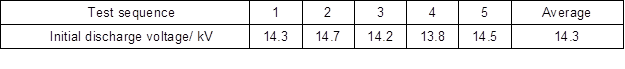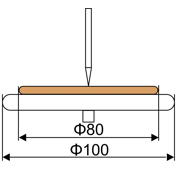Fig. 1. Needle plate discharge device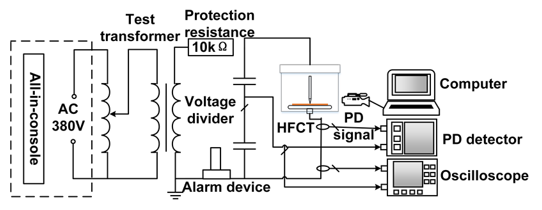Fig. 2. Discharge experimental platform

Image Segmentation with Watershed Algorithm

The main purpose of extracting fibre profiles from SEM images is to find segmentation points of fibre profiles and their background. Due to their similar colour, common segmentation methods cause over-segmentation; thus, the watershed image segmentation algorithm was selected based on a top-hat transformation in this research.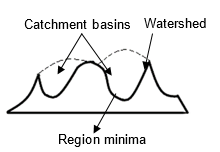Fig. 3. Schematic diagram of the watershed segmentation algorithm

The watershed segmentation algorithm is shown in Fig. 3. The aim is to treat each SEM image as a topological geomorphology map. Each pixel grey value is the elevation of that point; the local minimum in an area represents the catchment basin; and the surrounding boundary represents the watershed. It is assumed that a small hole is punctured in the catchment basin, and water is injected continuously from the hole. With increasing depth, the area of influence of each local minimum gradually expands outward, forming a watershed at the junction of the two collection basins (Vincent and Soille 1991). The gradient function is as follows,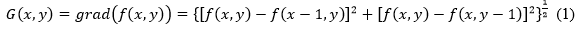where represents the original grey-scale image, and represents the gradient operation, since the individual watershed segmentation algorithm is prone to over-segmentation when extracting an unknown subject line. Although the boundary between fibre and background is more obvious, the fibres are of similar colour; therefore, it is necessary to split the image twice. In the first segmentation process, the OTSU algorithm can be used to segment the fibres from the background. The second time, a top-hot based watershed segmentation algorithm is applied to segment the fibres.

The OTSU (Du et al. 2019) is an algorithm for obtaining a binary image with which to segment the threshold. The main principle is to use the characteristics of the grey image to divide the picture into two parts: background and foreground. Setting the segmentation threshold of fibre and background as , in the binarised image, the ratio of the number of pixels of the fibre to the entire image is , the average grey-scale is , the ratio of the number of pixels in the background to the total number of pixels is , the background average grey-scale is , the average grey level of the image is , and the class square error is recorded as , the pixel points of the image length and width are and respectively, then: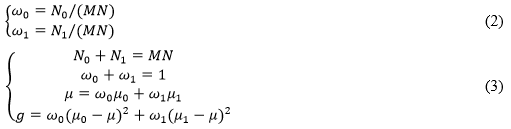If the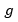is maximised, the threshold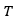is the required value, and the first divided image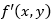is obtained.

Then, the watershed segmentation algorithm is used for the second step: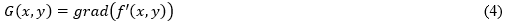Finally, the top-hat opening operation is used to operate the watershed image, selecting the appropriate structure b to operate on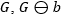for erosion operation,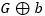for dilation operation,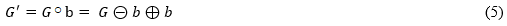where b is composed of three basic structural elements: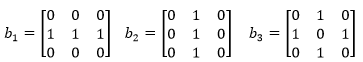(6)

Substituting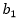,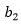, and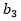into Equation 5 to obtain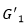,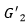, and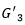, and assigning different weights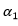,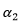, and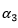to obtain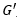, which is the final fibre extraction image: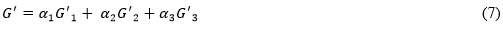The segmentation results of original SEM images (Fig. 4a) using the above segmentation algorithm are shown in Fig. 4b.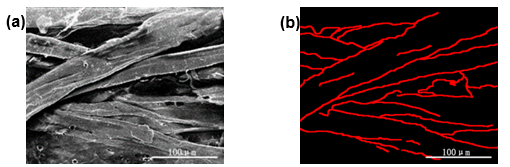Fig. 4. SEM micrograph of insulation pressboard: (a) The original image ×500, (b) Results of fibre contour image segmentation (marked resolution:397×314 Pixels)

Measurement Parameters

White marks area

To study the discharge characteristics of the surface discharge of the insulation pressboard under the needle plate model, the surface discharge white marks area of the insulating pressboard was selected as one of the discharge development criteria. The extent to which the tip discharge is destructive to the surface of the insulation pressboard could be determined by considering the white marks area. According to the image processing method used here, after adjusting the pressboard photos uniformly to 256 × 256 pixels, the white marks area was determined from images of the pressboard and the proportional area of the white marks was calculated.

In image processing, each picture is composed of pixels. Firstly, the RGB image was pre-processed to increase the colour contrast of the white marks and the pressboard, so that the white mark edge was more obvious. Secondly, it was converted into a grey-scale image. The colour distribution of the pixels in the grey-scale image is 0 to 255. When the grey value is 0, the pixel appears black, and when the grey value is 255, it appears white. After using image processing to improve the contrast, the white marks in the grey-scale image contrasted with the surrounding pressboard. Using MATLAB™ software, and setting a threshold T, the grey-scale image was further converted into a black and white image. Finally, using an editing program to calculate the total number of pixels and the number of white pixels in the picture, which were denoted as S and s respectively we calculated the ratio of the number of white marks pixels in the whole picture at each stage: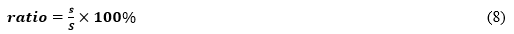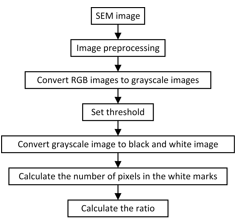Fig. 5. White marks area calculation flow chart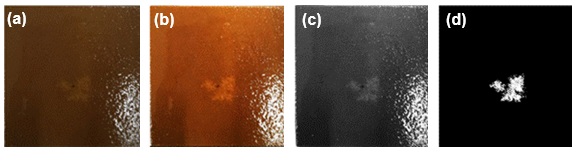Fig. 6. Insulating pressboard white mark extraction: (a) The original image, (b) Improve contrast, (c) Grayscale image, (d) Black and white graph

Fibre width

Most researchers use the MATLAB™ tool-kit to measure the diameter, but this method is inaccurate. The pressboard fibre tends to be curved and the thickness is unevenly distributed. Therefore, it is necessary to find the correct direction of the fibre, use this as the axis to measure the width in multiple places, and obtain the average value. The measurement flow chart is as shown in Fig. 7. To detect the fibre width, the aforementioned watershed segmentation algorithm based on a top-hat function is used to segment the image and extract the fibre contour (e.g., Fig. 8).

The specific steps for measuring the width using the central axis method are as follows: the first step is to determine the direction and choose a point next to the outline, denoted as . Second, take as the starting point, plot straight lines along the and directions, and observe the intersection of the two straight lines and the fibre outline. If the distance between the two intersections in the direction is greater than that in the direction, the fibre is measured in the -direction and vice versa. As shown in Fig. 9a, it is seen that the fibre should be measured in the direction.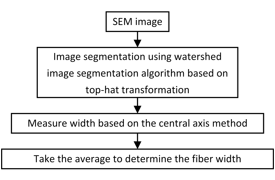Fig. 7. The flow chart of fibre width measurement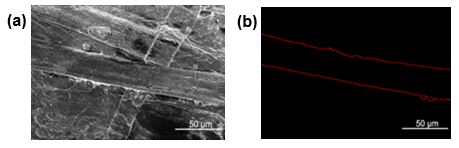Fig. 8. SEM micrograph of insulation pressboard: (a) insulating pressboard SEM original image×1000, (b) insulating pressboard fibre edge extraction map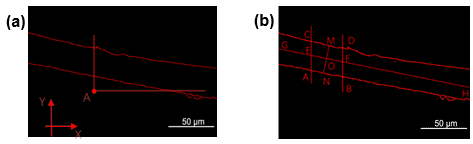Fig. 9. Central axis method for measuring fibre width: (a) Determine direction, (b) Measure width

Two points were chosen. These were close to each other on the fibre, and a straight line was plotted in the-direction, intersecting the fibre at , . The points and were connected. Their mid-points , respectively were found and connected . Finally, the mid-point of was determined. Then was passed as the vertical line of , and the fiber contour was crossed to . It was assumed that , that is, the width of a fibre. These steps were repeated many times, calculating , and taking the average value thereof.

Porosity

When using SEM images to observe the fibres, researchers seldom have observed the internal cross-section of the insulation pressboard. Here, the cross-sectional pore profile was extracted by the above segmentation algorithm and the porosity in different discharge states was analysed.

Figure 11a shows the cross-sectional image of the insulating pressboard, and the pores appear black on the image, which provides a contrast with the colour of the fibre. According to this feature, the porosity of the material can be calculated where is the porosity, is the pore area (the number of black pixels), and is the product of the cross-sectional area: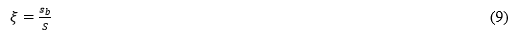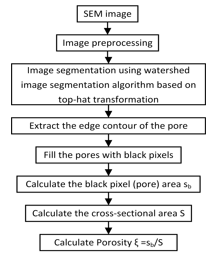Fig. 10. Porosity calculation flow chart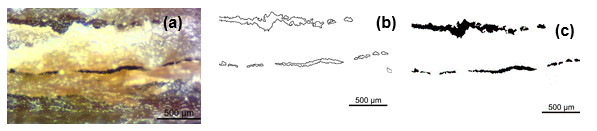Fig. 11. Insulating pressboard cross-section pore extraction diagram: (a) Cross-section of insulating pressboard, (b) Extract the edge of the insulating pressboard cross section, (c) Filled insulation pressboard cross section pore

The process involves adjusting the contrast and colour saturation of the image, pre-processing it to make the pore-fibre boundary clearer. Then the top-hat based watershed segmentation algorithm was used to segment the image to extract the pore boundary. Subsequently, the pores were filled with black pixels, thus obtaining the pore area ” ” (that is, the number of black pixels) and the cross-sectional area ” “, so as to obtain the porosity.

3D RECONSTRUCTION OF INSULATION PRESSBOARD FIBRE

The 3-D reconstruction of material microstructure combines image processing and computer technologies. The 2-D information about the material can be obtained by computer, and then the 3-D spatial model of material can be reconstructed.

As the SEM pictures can only show the surface of the insulation pressboard fibre morphology, the inner layer of the pressboard cannot be observed. To observe the internal microscopic morphology of the insulating pressboard, the complete sequence cross-section image of the sample was obtained by polishing the cross-section of the pressboard and reconstructing the associated 3-D model.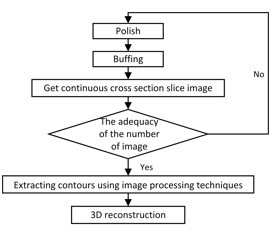Fig. 12. Flowchart for 3-D reconstruction of insulation pressboard fibre

The process was as follows:

1) Cut the pressboard into 2 mm × 10 mm × 10 mm cuboids (length × width × thickness).

2) Place the sample under an optical microscope and use a point on the edge as a fixed observation point.

3) 5000 mesh emery paper is used to polish the pressboard along the cross-section. After each polishing stage, measure the thickness of the sample block with a micrometre, and control the thickness of the polish to about 10 ± 2 μm.

4) Buff the sample block, and obtain the cross-sectional images of this fibre bundle layer under the microscope (Miao et al. 2017).

5) Repeat Steps 2 to 4 to obtain 100 consecutive cross-sectional slice images.

6) Pre-process the original image, by, for example: contrast expansion, image noise reduction, etc.

7) Extract the contour of the fibre to be extracted using the aforementioned segmentation algorithm.

8) Repeat Steps 6-7 to extract the desired fibre profile from 100 cross-sectional images.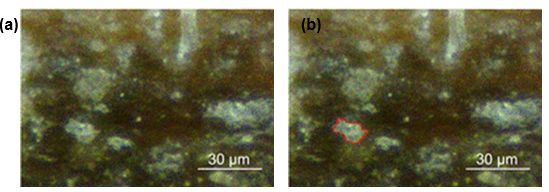Fig. 13. Insulated pressboard cross section image: (a) Insulated pressboard cross section image, (b) Pressboard cross-section fibre profile extraction

9) Pre-process the contours. Due to problems such as placement of the sample under the microscope and the accurate focussing thereof, the centre position of the adjacent two cross-section fibre outlines may vary greatly. Therefore, it is necessary to make the centre points of adjacent contour lines coincide so that the size ratio is the same (Eqs. 9 and 10) (Ma et al. 2007). The specific approach is to assume that the fibre profiles of adjacent cross sections are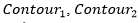. The coordinates of the center point are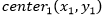,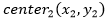, width and height are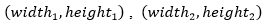, the translation distance and scaling factor of the profile are and , respectively. Then, the coordinates of each point on the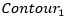are kept unchanged, and the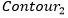coordinates are adjusted using the following equation.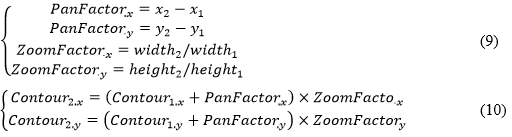Construct a triangular mesh model using the shortest diagonal method. Assume that the pixel points of the fibre profiles in two adjacent cross-sections are, respectively,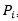,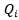, and call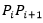and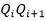the line segment of the contour. If the two endpoints of a contour line segment are connected to a point on the contour line of the adjacent cross section, it forms a triangular face. The so-called 3-D reconstruction with contour is to connect the contour lines of two surfaces with a series of triangular faces. Since the contour has been pre-processed, the shortest diagonal method can be used to construct the triangular mesh model, the shortest distance between two adjacent contour points can be calculated by the following equation: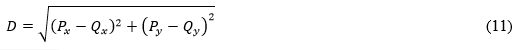where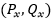are the coordinates of the current point, and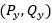are the coordinates closest to the current point. In this method, all contour lines are connected to form a triangular mesh model.

11) Render the triangular mesh model and complete the reconstruction.

Figure 14 shows the 3-D model of the fibres. The construction of the 3-D model of a fibre overcomes the limitation of a 2-D image where only surface fibres can be observed. Using 3-D reconstruction techniques, fibres can be more clearly observed from different angles to see the contours of the fibre, the surface roughness, internal holes, and staggered distributions thereof.